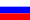# Vestnik KRAUNC. Fiz.-Mat. Nauki. 2022. vol. 40. no. 3. pp. 64–71. ISSN 2079-6641

Contents of this issue

Read Russian VersionMSC 26A33, 34B05

Research Article

Solution of a mixed boundary value problem for an equation with fractional derivatives with different origins

L. M. Eneeva

Institute of Applied Mathematics and Automation, Kabardino-Balkarian Scientific Center RAS, 360000, Nalchik, Shortanova st., 89 A, Russia
E-mail: eneeva72@list.ru

A solution of a mixed boundary value problem for an ordinary differential equation of fractional order is constructed. The main part of the equation is a composition of left- and right-hand Riemann-Liouville and Caputo fractional differentiation operators. A representation of the solution of the problem under study is found,
and an estimate for the eigenvalues is also obtained.

Key words: fractional differential equation with different origins, mixed boundary value problem, Riemann-Liouville derivative, Caputo derivative.

DOI: 10.26117/2079-6641-2022-40-3-64-71

Original article submitted: 26.10.2022

Revision submitted: 23.11.2022

For citation. Eneeva L.M. Solution of a mixed boundary value problem for an equation with fractional derivatives with different origins. Vestnik KRAUNC. Fiz.-mat. nauki. 2022, 40:3, 64-71. DOI: 10.26117/2079-6641-2022-40-3-64-71

Competing interests. The author declares that there are no conflicts of interest regarding authorship and publication.

Contribution and Responsibility. The author contributed to this article. The author is solely responsible for providing the final version of the article in print. The final version of the manuscript was approved by the author.

Funding. The work was carried out within the framework of the state assignment (state registration number No. 122041800015-8)

References

1. Nakhushev A. M. Drobnoye ischisleniye i yego primeneniye [Fractional calculus and its application]. Mjscow, Fizmatlit, 2003. 272 (In Russian).
2. Rekhviashvili S. Sh. Lagrange formalism with fractional derivative in problems of mechanics, Technical Physics Letters, 2004, 30:2, 33–37.
3. Rekhviashvili S. Sh. Fractional derivative physical interpretation, Nonlinear world, 2007, 5:4, 194–197 (In Russian).
4. Eneeva L. M. Boundary value problem for differential equation with fractional order derivatives with different origins, Vestnik KRAUNC. Fiz.-Mat. Nauki, 2015, 2(11), 39–44,(In Russian).
5. Eneeva L. M. An estimate for the first eigenvalue of the dirichlet problem for an ordinary differential equation with fractional derivatives with different origins, News Of The Kabardino-Balkarian Scientific Center Of RAS, 2017, 1(75), 34–40 (In Russian).
6. Eneeva L. M. On Neumann problem for equation with fractional derivatives with different starting points, Vestnik KRAUNC. Fiz.-Mat. Nauki, 2018, 4(24), 61–65 (In Russian).
7. Eneeva L. M. Lyapunov inequality for an equation with fractional derivatives with different origins, Vestnik KRAUNC. Fiz.-Mat. Nauki, 2019, 28:3, 32–40 (In Russian).
8. Eneeva L. M. A priori estimate for an equation with fractional derivatives with different origins, Vestnik KRAUNC. Fiz.-Mat. Nauki, 2019, 29:4, 41–47 (In Russian).
9. Eneeva L. M., et. al. Lyapunov inequality for a fractional differential equation modelling damped vibrations of thin film MEMS, Advances in Intelligent Systems and Computing. ICCD2019 (paper ID: E19100).
10. Rekhviashvili S. Sh., et.al. Modeling damped vibrations of thin film MEMS, Advances in Intelligent Systems and Computing. ICCD2019 (paper ID: E19101).
11. Stankovi´c B. An equation with left and right fractional derivatives, Publications de l’institut math´ematique. Nouvelle s´erie, 2006, 80(94), 259–272.
12. Atanackovic T. M., Stankovic B. On a differential equation with left and right fractional derivatives, Fractional Calculus and Applied Analysis, 2007, 10:2, 139–150.
13. Torres C. Existence of a solution for the fractional forced pendulum, Journal of Applied Mathematics and Computational Mechanics, 2014, 13:1, 125–142.
14. Tokmagambetov N., Torebek B. T. Fractional Analogue of Sturm-Liouville Operator, Documenta Mathematica, 2016. 21, 1503–1514.
15. Eneeva L., Pskhu A., Rekhviashvili S. Ordinary Differential Equation with Left and Right Fractional Derivatives and Modeling of Oscillatory Systems. Mathematics, 2020. 8(12), 2122.
16. Eneeva L. M. Mixed boundary value problem for an ordinary differential equation with fractional derivatives with different origins, Vestnik KRAUNC. Fiz.-Mat. Nauki, 2021, vol. 36:3, 65–71.
17. Pr¨odorf S. Linear integral equations, Analysis – 4, Itogi Nauki i Tekhniki. Ser. Sovrem. Probl. Mat. Fund. Napr., 1988. 27, 5–130.

Eneeva Liana Magometovna – Ph. D. (Phys. & Math.), Senior Researcher at the Institute of Applied Mathematics and Automation of the Kabardino-Balkarian Scientific Center of the Russian Academy of Sciences, Nalchik, Russia, ORCID 0000-0003-2530-5022.